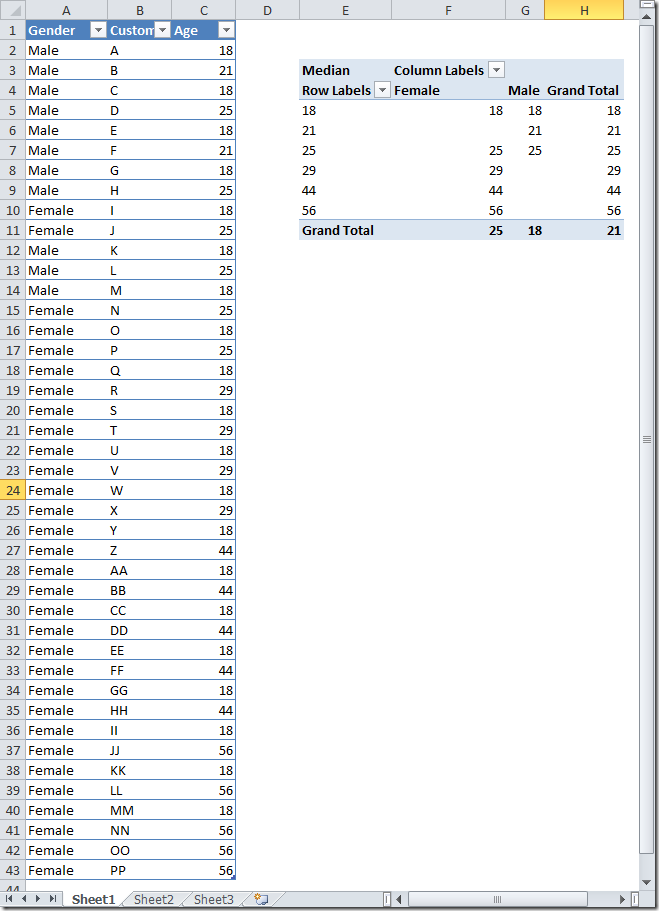I recently discussed with Darren Gosbell about how to calculate the Median in DAX.

The problem is to make the calculation using a measure, so that you can use it in a dynamic way into a PivotTable. We have a People table with three columns: Gender, Customer and Age (you can see the table in the screenshot below). The Median formula can be defined in this way:

MINX( FILTER( VALUES( People[Age] ),
CALCULATE( COUNTROWS( People ),
People[Age] <= EARLIER( People[Age] ) )
> COUNTROWS( People ) / 2 ),
People[Age] )

The MINX try to find the minimum Age value which has been filtered by the FILTER condition.
The FILTER enumerates all the ages and, for each, CALCULATE the number of people that have an age lower than or equal to the “current” one (here the concept of current is returned by EARLIER, which refers to the row context outside the CALCULATE). The FILTER returns only those ages for which the previous CALCULATE is at least half of the whole population.

I don’t like very much that MINX also calculates the same value for values of Ages that are higher to the lower number that can be found. But this is a logic that applies to an algorithm that iterates data in a single thread. I don’t see any reason why the MINX couldn’t be executed in parallel, and I’d like to know if this is what really happens under the cover – more info if I will discover something.

Below the screenshot of the example and the PivotTable with the calculated Median.MINXReturns the smallest value that results from evaluating an expression for each row of a table. Strings are compared according to alphabetical order.

MINX ( <Table>, <Expression> )

FILTERReturns a table that has been filtered.

FILTER ( <Table>, <FilterExpression> )

VALUESWhen a column name is given, returns a single column table of unique values. When a table name is given, returns a table with the same columns and all the rows of the table (including duplicates) with the additional blank row if present.

VALUES ( <TableNameOrColumnName> )

CALCULATEContext transition

Evaluates an expression in a context modified by filters.

CALCULATE ( <Expression> [, <Filter> [, <Filter> [, … ] ] ] )

COUNTROWSCounts the number of rows in a table.

COUNTROWS ( [<Table>] )

EARLIERReturns the value in the column prior to the specified number of table scans (default is 1).

EARLIER ( <ColumnName> [, <Number>] )